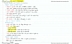# Economics 1022A/B Study Guide - Final Guide: Aggregate Demand, Monetary Base, Chapter 27

249 views3 pagesmaroonwoodchuck8495771 and 39243 others unlocked27
Verified Note
27 documents

## Document Summary

M a c r o e c o n o m i c s 1 0 2 2. Gdp expidenture approach = c + i + g + nx. = gdp income approach (+ statistical discrepancy = Growth rates = (new value initial) / (initial) Unemployment rate = (total # of unemployed / labor force) *100. Ratio = (total # of employed / working age population) *100. Involuntary part time rate = (# of inv. Rate = (labor force / working age population) *100. Consumer price index = (cpi basket @ current year prices) * 100 (cpi basket @ base year prices) Inflation rate = cpi (cy) cpi (by) *100. Gdp deflator = (nominal gdp / real gdp) * 100. Chained price index for consumption = nominal cons. Real gdp per person = rgdp / population. Labor productivity = rgdp / aggregate labor hours. Interest rate = \$ of interest / investment.